## 变换群

### 变换群与单参数变换群

#### 变换群

$X$ 是一个光滑流形。若 $\Phi : X \rightarrow X$ 是一个微分同胚，则称 $\Phi$$X$ 的一个 变换。我们可将 $X$ 的所有变换形成的集合记为 $\mathrm{Diff}(X)$。以映射的复合为乘法，$\mathrm{Diff}(X)$ 形成一个群（恒元为恒等映射，逆映射存在保证逆元存在）。显然对于 $X$ 的一个变换，我们可以定义其在流形的光滑张量场上的推前和拖回映射 $\Phi_*$$\Phi^*$

$\Phi : G \times X \rightarrow X ,\quad (g,p) \mapsto \Phi(g,p)$

$\Phi_g : X \rightarrow X ,\quad p \mapsto \Phi_g (p) = \Phi(g,p)$

$\Phi_g$ 满足：

• $\Phi_e$ = $\mathrm{Id}_X$，其中 $e$$G$ 的恒元
• $\Phi_{gh} = \Phi_g \circ \Phi_h ,\ \forall g,h \in G$（或 $\Phi_{hg} = \Phi_g \circ \Phi_h ,\ \forall g,h \in G$）。

$G\rightarrow \mathrm{Diff}(X),\quad g\rightarrow \Phi_g\in \mathrm{Diff}(X)$

$\Phi : G \times X \rightarrow X$ 是一李氏变换群，则称 $\{\Phi(g,p)|g \in G\}$ 为群过 $p \in X$ 点的轨道，其中 $g$ 跑遍整个群 $G$

$\Phi : G \times X \rightarrow X$ 是一李氏变换群，则称 $\{p| \Phi(g,p) = p,\ \forall g \in G\}$ 为该群作用的固定点集。

$\Phi : G \times X \rightarrow X$ 是一李氏变换群，则称 $\{g| \Phi(g,p) = p\}$ 为该群作用在 $p$ 的迷向子集。

• $G$$X$ 上的作用称为 有效的（effective），若对 $\forall p\in X,\ \Phi_g (p) = p$ 意味着 $g = e$
• $G$$X$ 上的作用称为 自由的（free），若除了 $g = e$ 之外，$\Phi_g (p)\neq p$
• $G$$X$ 上的作用称为 可迁的（transitive），若对任意 $p,q \in X$，总存在一个 $g \in G$ 使得 $\Phi_g (p) = q$

#### 单参数变换群

$\Phi : \mathbb{R} \times X \rightarrow X ,\quad (t,p)\mapsto \Phi(t,p)$

$\Phi_t : X \rightarrow X ,\quad p \mapsto \Phi_t (p) = \Phi(t,p)$

$\Phi_t$ 满足：

• $\Phi_0 = \mathrm{Id}_X ,$
• $\Phi_{t+s} = \Phi_t \circ \Phi_s ,\ \forall t,s \in R$

$\Phi_p: \mathbb{R} \rightarrow X,\quad t\mapsto \Phi_p(t) = \Phi(t,p)$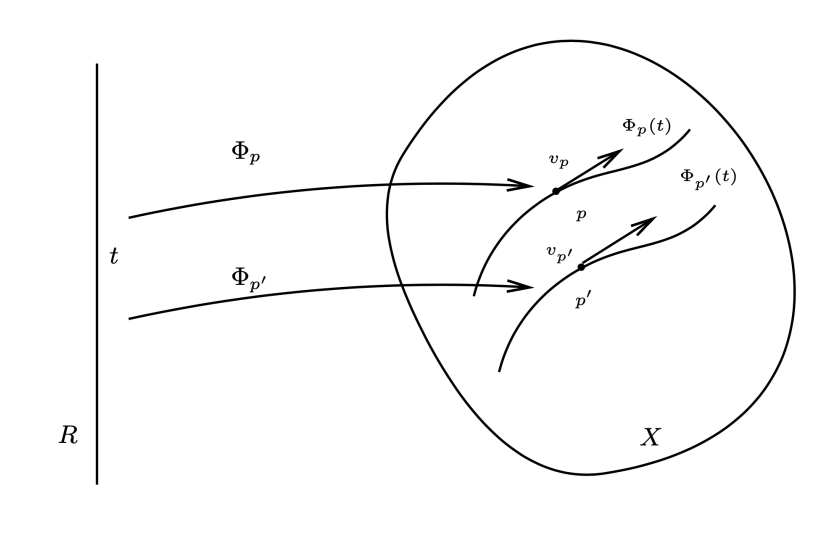$\Phi_t$ 可表示为：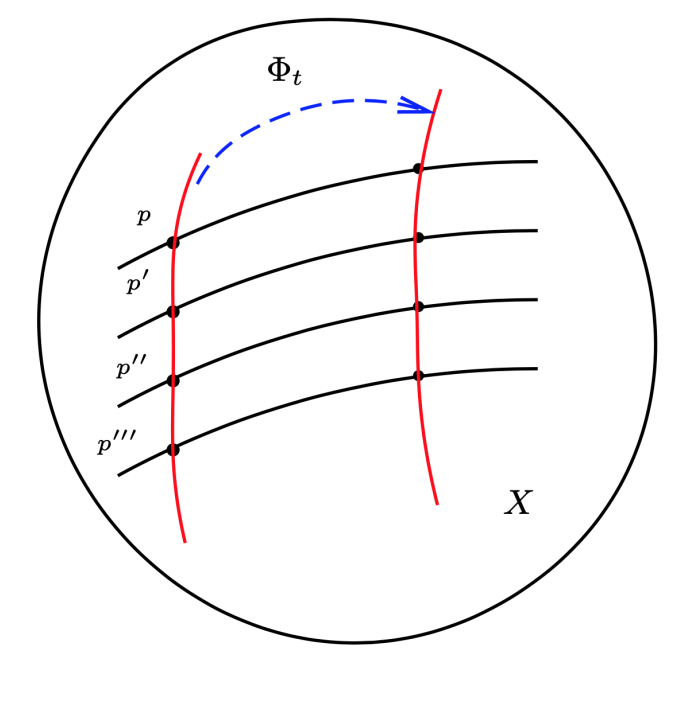$v_p=\frac{d(\Phi_p(t))^i}{dt}|_ {t=0} \frac{\partial }{\partial x^i}| _ {p}$

$q = \Phi_p(s) = \Phi(s,p)$，则我们有：

\begin{aligned} \Phi_q(t) &= \Phi(t,q) = \Phi(t,\Phi(s,p)) = \Phi_t\circ \Phi_s(p)\\ &= \Phi_{t+s}(p) = \Phi(t+s,p) = \Phi_p(t+s) \end{aligned}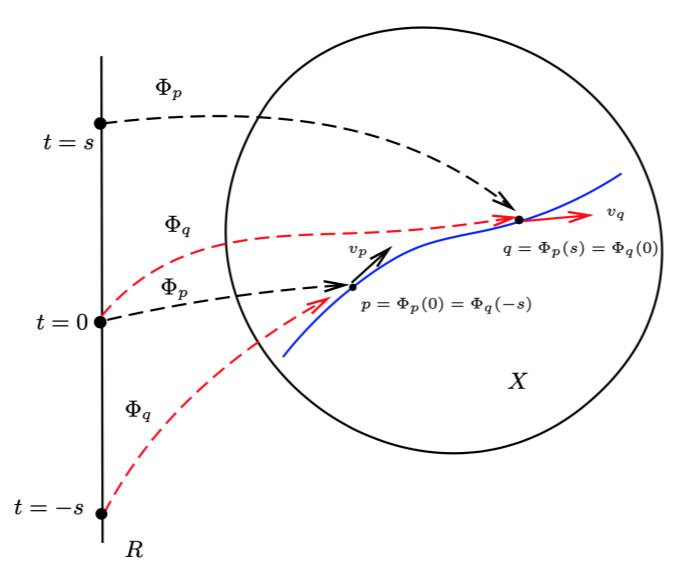\begin{aligned} v_q(f) &= \frac{d (f\circ \Phi_{q}(t))}{dt}|_{t=0}\\ &= \frac{d(f\circ \Phi_p(t+s))}{dt}|_{t=0}\\ &= \frac{d(f\circ \Phi_p(t))}{dt}|_{t=s}\\ \end{aligned}

\begin{aligned} v_q(f)&=\frac{d (f\circ \Phi_{p}(t+s))}{dt}|_{t=0}\\ &=\frac{d (f\circ \Phi_{s}\circ \Phi_p(t))}{dt}|_{t=0}\\ &=v_p(f\circ \Phi_s)\\ &=v_p((\Phi_s)^*(f))\\ &=(\Phi_s)_*v_p(f) \end{aligned}

$(\Phi_s)_*v_p = v_{\Phi_s(p)}$

$v$ 是光滑流形 $X$ 上的光滑矢量场，而 $\Phi:X\rightarrow X$ 是一个变换，若 $\Phi_*v = v$，则称 $v$ 在变换 $\Phi$ 下不变，称为 不变矢量场。上式表明：单参数变换群的诱导切矢量场 $v$ 在单参数变换 $\{\Phi_t,t\in\mathrm{R}\}$ 下不变。

#### 李群的单参数子群

$\mathbb{R} \rightarrow G, t \mapsto g(t)$

$g(t)g(s) = g(t + s),\quad g(0) = e$

Killing 矢量场
$G$ 是左作用在 $X$ 上的李群, 即我们有李变换群 $\{\Phi g ,g \in G\}$ 李群 $G$ 的单参数子群生给出一个 $X$ 上的单参数变换群

$\{\Phi_{g(t)} ,t \in R\} \subset \mathrm{Diff}(X)$

#### 局部单参数变换群

$U$ 是光滑流形 $X$ 的一个开集。若光滑映射

$\Phi : (-\epsilon,\epsilon) \times U \rightarrow X ,\quad \epsilon > 0$

• $\Phi_0 = \mathrm{Id}_U$
• 如果 $|s|,|t|,|t + s| < \epsilon$$p,\Phi_t (p) \in U$，存在

$\Phi_ s \circ \Phi_t(p) = \Phi_ {t+s} (p)$

$\Phi_t (p) = \Phi(t,p)$

$v$ 是光滑流形 $X$ 上的光滑切矢量场，则对于任意一点 $p \in X$，存在一个邻域 $N(p)$ 和一个作用在 $N(p)$ 上的局部单参数变换群 $\{\Phi_t ,|t| < ϵ\}$ 使得矢量场 $v$$N(p)$ 上的限制，即：$v|_ {N(p)}$ 是这个局部单参数变换群的诱导切矢量场。

$X$ 是紧致的光滑流形，则 $X$ 上的任意一个光滑切矢量场生成一个单参数变换群。即存在一个单参数变换群使得它的诱导切矢量场恰好是这个给定的切矢量场。

### 李导数

$v$$u$ 是光滑流形 $X$ 上的两个光滑矢量场。$v$$p$ 点的邻域 $N(p)$ 生成的局部单参数变换记为 $\Phi_ t$。切矢量场 $u$ 沿着切矢量场 $v$$p$ 点的李导数定义为：

$\mathcal{L}_vu| _ p=\lim_{t\rightarrow 0}\frac{(\Phi_{-t})_ * u_{\Phi_{t}(p)}-u_p}{t}$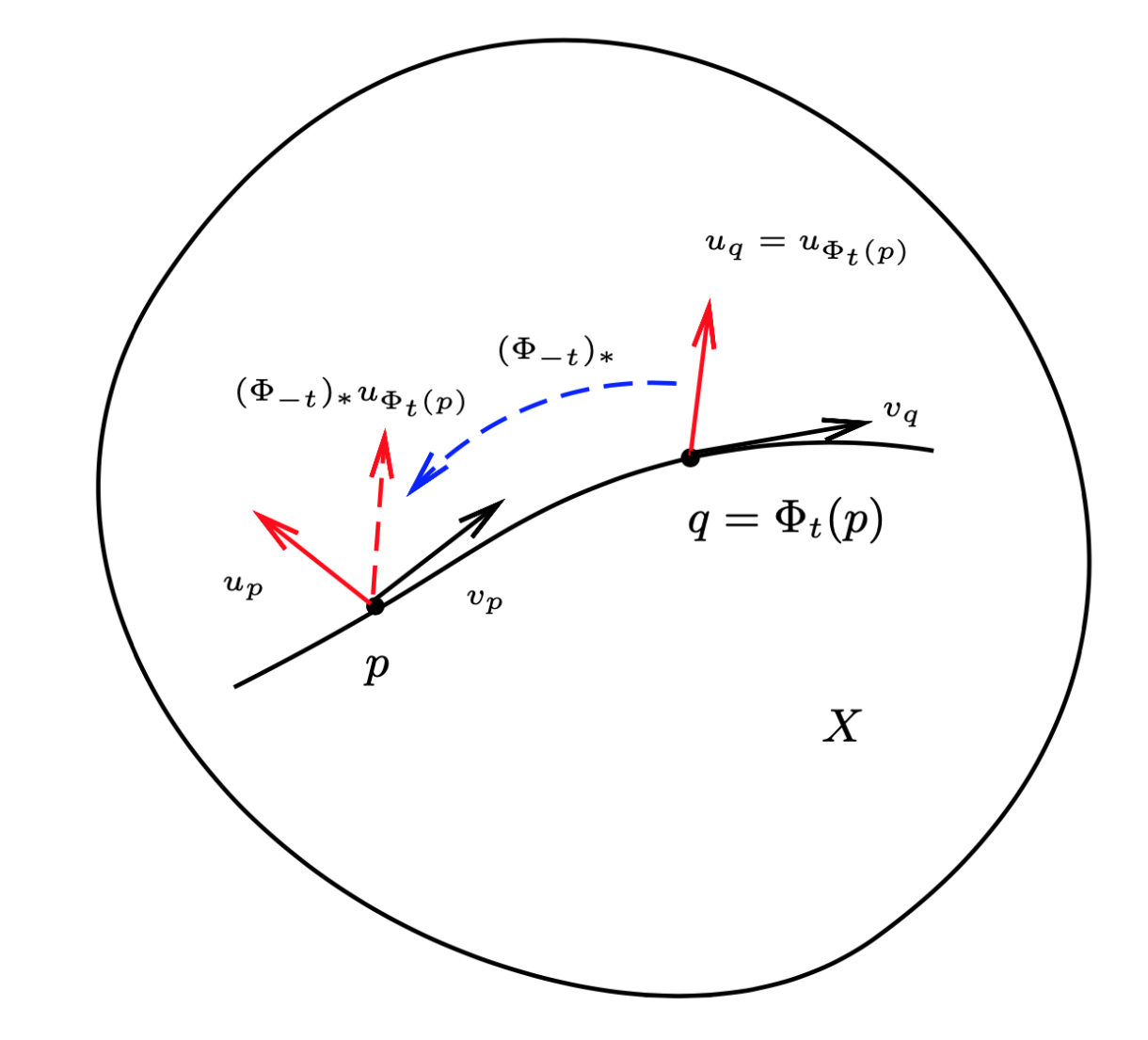$\mathcal{L}_v u| _ p = [v,u]|_ p .$

$\mathcal{L}_vu=\lim_{t\rightarrow 0}\frac{(\Phi_{-t}) _ * u-u}{t}=[v,u]$

$\mathcal{L}_v: \mathscr{X}(X) \rightarrow \mathscr{X}(X)$

\begin{aligned} v(f)|_{p} &= \frac{d(f\circ \Phi_p(t))}{dt}|_{t=0}\\ &= \frac{d(f\circ \Phi_t(p))}{dt}|_{t=0}\\ &= \frac{d}{dt}((\Phi_t)^*f)(p)|_{t=0}\\ &=\lim_{t\rightarrow 0} \frac{((\Phi_t)^*f)(p)-f(p)}{t}\\ &=\mathcal{L}_{v}f\\ \end{aligned}

$\tau$$s-$ 阶协变张量场，$v \in \mathscr{X} (X)$，而 $\Phi_ t$ 是由 $v$ 生成的局部单参数变换。则 $\tau$ 关于切矢量场 $v$ 的李导数定义为：

$\mathcal{L}_v\tau=\lim_{t\rightarrow 0}\frac{(\Phi_t)^* \tau-\tau}{t}$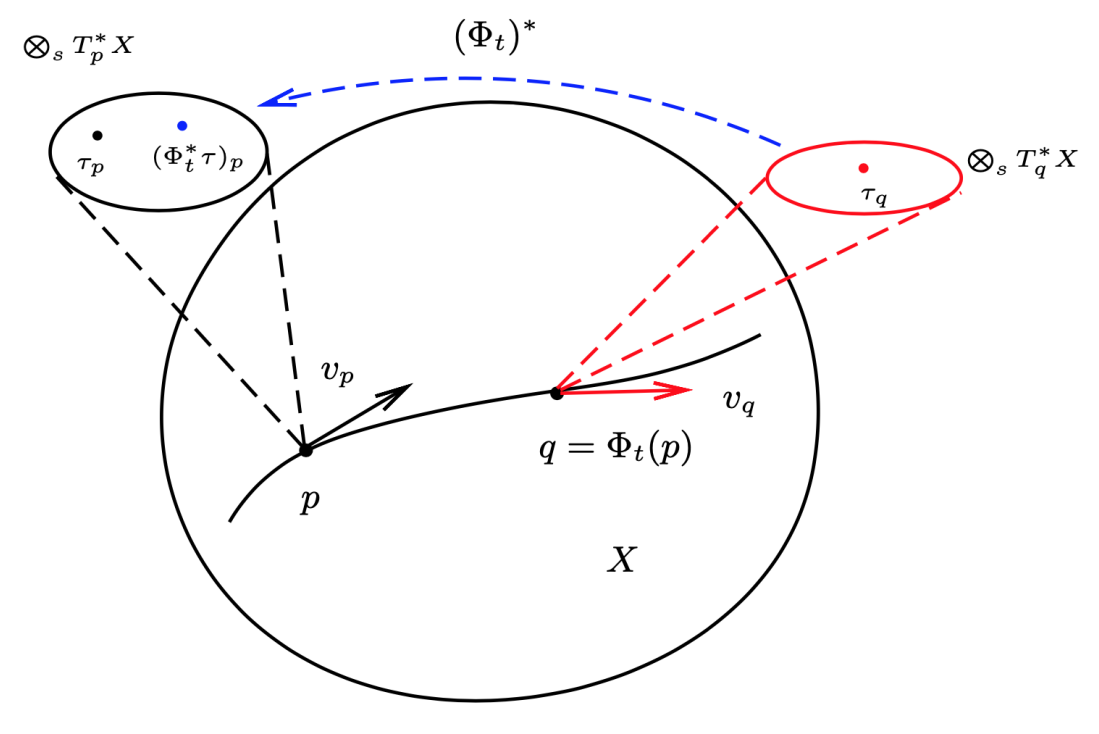$\tau$$s$-阶逆变张量场，$v \in X (X)$，而 $\Phi_t$ 是由 $v$ 生成的局部单参数变换. 则 $\tau$ 关于切矢量场 $v$ 的李导数定义为：

$\mathcal{L}_v\tau=\lim_{t\rightarrow 0}\frac{(\Phi_{-t})_ * \tau-\tau}{t}$

$\mathcal{L}_v\tau=\lim_{t\rightarrow 0}\frac{(\Phi_t)^* \tau-\tau}{t}$

• $\mathbb{R}-$ 线性：$\forall \lambda_ 1 ,\lambda_ 2\in \mathbb{R}$，相同类型的张量 $\tau_ 1 , \tau_ 2$ 我们有

$\mathcal{L}_v (\lambda_ 1 \tau_ 1 + \lambda_ 2 \tau_ 2 ) = \lambda_ 1 \mathcal{L}_ v \tau_ 1 + \lambda_ 2 \mathcal{L}_ v \tau_ 2$

• Leibniz 法则：设 $\tau_ 1$$\tau_ 2$ 是两个张量场，它们的类型可以不同。我们有：

$\mathcal{L}_ v (\tau_ 1 \otimes \tau_ 2 ) = (\mathcal{L}_ v \tau_ 1 ) \otimes \tau_ 2 + \tau_ 1 \otimes(\mathcal{L}_ v \tau_ 2)$

• 和缩并可交换：

$\mathcal{L}_ v \circ C(\tau) = C \circ \mathcal{L}_ v (\tau)$

$f\in \mathscr{F}(X)$，得到：

$\mathcal{L}_vf = v(f) = v^i\frac{\partial f}{\partial x^i}$

$\mathcal{L}_v\frac{\partial}{\partial x^i}=[v,\frac{\partial}{\partial x^i}]=-\frac{\partial v^j}{\partial x^i}\frac{\partial}{\partial x^j}$

$C\circ \mathcal{L}_v(dx^i\otimes \frac{\partial}{\partial x^j}) = \mathcal{L}_v\circ C(dx^i\otimes \frac{\partial}{\partial x^j}) = \mathcal{L}_v \delta^i_{\ \ j} = 0$

\begin{aligned} 0 &= C(\mathcal{L}_v dx^i\otimes \frac{\partial}{\partial x^j} + dx^i\otimes \mathcal{L}_v\frac{\partial}{\partial x^j})\\ &= C(\mathcal{L}_v dx^i\otimes \frac{\partial}{\partial x^j} - \frac{\partial v^k}{\partial x^j} dx^i\otimes \frac{\partial}{\partial x^k})\\ \end{aligned}

$C(\mathcal{L}_v dx^i\otimes \frac{\partial}{\partial x^j}) = \frac{\partial v^i}{\partial x^j}$

$\mathcal{L}_v dx^i = A^i_{\ \ k}dx^k$，则由上式得到：

$A^i_{\ \ j} = \frac{\partial v^i}{\partial x^j}$

$\mathcal{L}_v dx^i=\frac{\partial v^i}{\partial x^j}dx^j$

$u,v \in \mathscr{X} (X),\ f \in \mathscr{F}(X)$， 则有：

• $\mathcal{L}_ {u+v} = \mathcal{L}_ {u}+\mathcal{L}_ {v}$
• $\mathcal{L}_ {[u,v]} = \mathcal{L}_ {u}\circ \mathcal{L}_ {v}-\mathcal{L}_ {v} \circ \mathcal{L}_ {u}$
• $\mathcal{L}_ {fv} u = [fv,u] = f\mathcal{L}_ v u - u(f)v$

### 微分同胚变换与坐标变换

• 主动观点（active viewpoint）：点发生变化，即 $\Phi : p \rightarrow \Phi(p)$，张量 $\tau_p$ 被映射到 $\Phi(p)$ 点的张量 $\Phi_{* p} \tau_p$ 。在这种观点下，$X$ 上的张量场 $\tau$ 在微分同胚变换下变成 $X$ 上的另外一个张量场 $\Phi_ {*} \tau$，即

$\tau \rightarrow \Phi_ * \tau$

• 被动观点（passive viewpoint）：
点没有发生变化，而认为 $\Phi$（对张量）的作用是对“张量分量” 做了一个坐标变换。张量 $\tau_ p$ 的分量在这个坐标变换后恰好等于主动观点中 $\Phi_ {*p} \tau_ p$ 的分量。在这一观点下，张量的分量是重要的。

$\mathscr{G}(\Phi_ * \tau ,\Phi_ * \sigma ,\cdots) = \Phi_ * \mathscr{G}(\tau ,\sigma ,\cdots),\quad\ \forall\Phi \in \mathrm{Diff(X)}$

$\mathscr{G}^{i_1\cdots i_m}_{\qquad j_1\cdots j_n}(\tilde{\tau}^{i_1\cdots i_r}_{\qquad j_1\cdots j_s},\tilde{\sigma}^{i_1\cdots i_p}_{\qquad j_1\cdots j_q},\cdots)=\tilde{\mathscr{G}}^{i_1\cdots i_m}_{\qquad j_1\cdots j_n}(\tau^{i_1\cdots i_r}_{\qquad j_1\cdots j_s},\sigma^{i_1\cdots i_p}_{\qquad j_1\cdots j_q},\cdots)$

$\mathscr{G}(\Phi_ * \tau ,\Phi_ * \sigma ,\cdots) = \mathscr{G}(\tau ,\sigma ,\cdots)$

$\Phi_* \tau = \tau\quad or\quad \Phi^* \tau =\tau$

$\tilde{\tau}^{i_1\cdots i_r}_{\qquad j_1\cdots j_s}=\tau^{i_1\cdots i_r}_{\qquad j_1\cdots j_s}$

$\Phi_ t$ 所对应的无穷小坐标变换可以写为：

$\tilde{x}^ i = x^ i + tv^i$

$\tilde{x}^ i = x^ i - tv^i$

$\mathcal{L}_v\tau = 0$

\begin{aligned} 0 =& v^i\partial_i\tau^{i_1\cdots i_r}_{\qquad j_1\cdots j_s}\\ &-(\partial_k v^{i_1})\tau^{ki_2\cdots i_r}_{\qquad j_1\cdots j_s}-(\partial_k v^{i_r})\tau^{i_1\cdots k}_{\qquad j_1\cdots j_s}\\ &+(\partial_{j_1} v^{l})\tau^{i_1\cdots i_r}_{\qquad lj_2\cdots j_s}-(\partial_{j_s} v^{l})\tau^{i_1\cdots i_r}_{\qquad j_1\cdots j_s-1l}\\ \end{aligned}